Definitions of Square Dance Calls and Concepts

Index -->  Plus  |  A1  |  A2  |  C1  |  C2  |  C3A  |  C3B  |  C4  |  NOL  |
Definitions (Text Only) -->  Plus  |  A1  |  A2  |  C1  |  C2  |  C3A  |  C3B  |  C4  |  NOL  |
 Find call:

 \$B8@8l(B

From Parallel Lines, Twin Diamonds, a Generalized Thar, or other applicable formations. EN: 10
\$BJ?9T\$J(B Line, Twin Diamonds, \$B0lHL2=\$5\$l\$?(B Thar, \$B\$^\$?\$OB>\$NE,@Z\$J(B formation \$B\$+\$i!%(B JP: 10

Centers \$B\$O(B Trade and Spread \$B\$r(B, Ends \$B\$O(B Crossover Circulate \$B\$r\$7\$^\$9!%(B JP: 20

Note: The dancer's verbal response to Trade The Deucey is 'Quack, Quack!!' EN: 30
\$BCmTrade The Deucey \$B\$N%@%s%5!<\$N@<\$N1~Ez\$O(B 'Quack, Quack!!' \$B\$G\$9!%(B JP: 30

Parallel Waves \$B\$+\$i(B: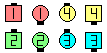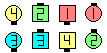Trade The Deucey\$B\$NA0(B \$B8e(B
Twin Diamonds \$B\$+\$i(B: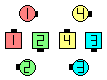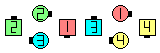Trade The Deucey\$B\$NA0(B \$B8e(B
Thar \$B\$+\$i(B: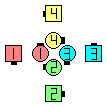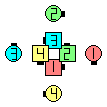Trade The Deucey\$B\$NA0(B \$B8e(B
Starting Double Pass Thru (Zero Tag) \$B\$+\$i(B: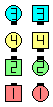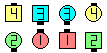Trade The Deucey\$B\$NA0(B \$B8e(B

Cheat: Acey Deucey & Spread. (This cheat does not work if the Ends start in Columns.) EN: 40
Cheat: Acey Deucey & Spread. (\$B\$3\$N(B cheat \$B\$O(B Ends \$B\$,(B Columns \$B\$+\$i;O\$a\$k\$H\$-\$K\$O;H\$(\$^\$;\$s(B.) JP: 40CALLERLAB definition for Trade The DeuceyChoreography for Trade The DeuceyComments? Questions? Suggestions?